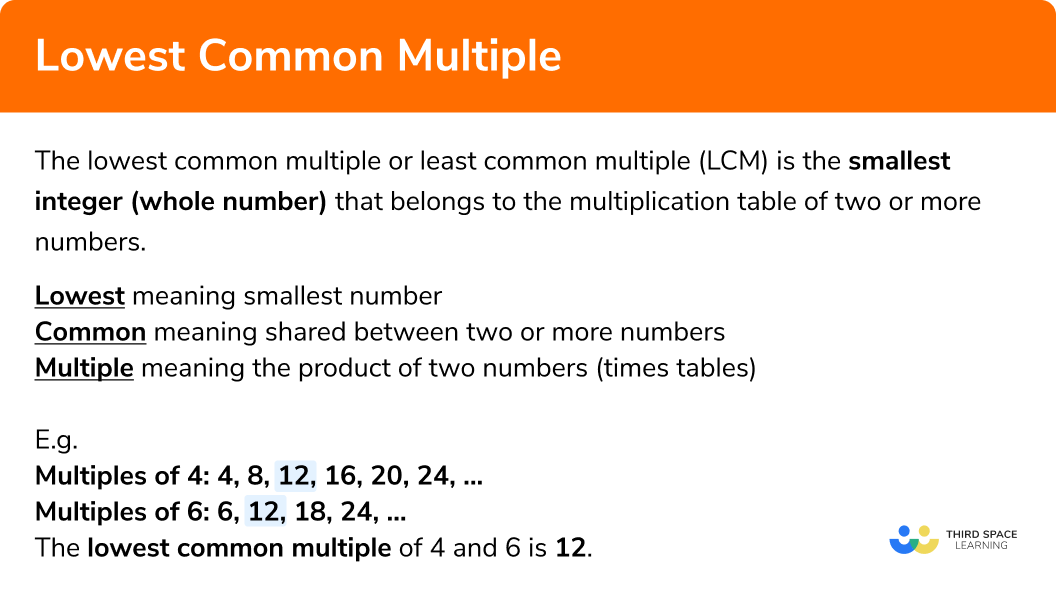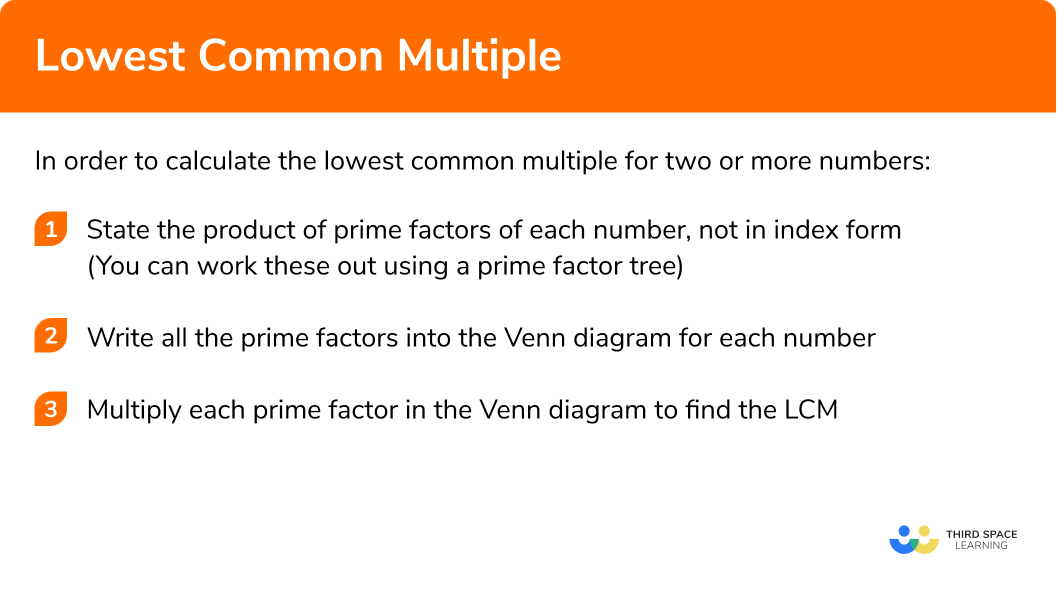# Lowest Common Multiple

Here we will learn about the lowest common multiple including how to calculate the lowest common multiple, use the lowest common multiple, and recognise when to calculate the lowest common multiple to answer complex worded problems.

Their are also lowest common multiple worksheets based on Edexcel, AQA and OCR exam questions, along with further guidance on where to go next if you’re still stuck.

## What is the lowest common multiple?

The lowest common multiple or least common multiple (LCM) is the smallest integer (whole number) that belongs to the multiplication table of two or more composite numbers.

Lowest meaning smallest number

Common meaning shared between two or more numbers

Multiple meaning the product of two numbers (times tables)

E.g.
Find the LCM of 4 and 6.

Let’s start by writing the multiples of 4 and 6

We can see that the smallest positive integer that occurs in each list is 12,
hence the lowest common multiple of 4 and 6 is 12.

NOTE: 24 occurs in both lists so is a common multiple, but not the lowest common multiple.

### What is the lowest common multiple?### Lowest common multiple and prime factors

Calculating the lowest common multiple becomes more complicated for larger numbers as listing the multiples of each number is very time consuming.

To make it easier we utilise the prime factors of the number.

The fundamental theorem of arithmetic states that each number greater than 1 is either a prime number or can be written by a product of primes.
This means that every number has a unique set of prime factors

We can use prime factors and a Venn diagram to calculate the lowest common multiple.
To do this we need to write out the prime factorisation of each number fully (without any powers) and then put the numbers into the Venn diagram by looking for pairs.

Here, we have the prime factors of 12 and 30.

• In the left circle are the prime factors of 12 (2, 2, and 3).
• In the right circle, we have the prime factors of 30 (2, 3, and 5).
• The dark orange section represents the prime factors of 12 that are not prime factors of 30 (which is just the prime factor of 2).
• The light orange section represents the prime factors of 30 that are not prime factors of 12 (which is just the prime factor of 5).
• The intersection represents the prime factors of 12 and 30 (2 and 3).
• The highest common factor for 12 and 30 is 2 × 3 = 6.

The lowest common multiple of 12 and 30 is equal to the product of all the factors viewed in the Venn diagram

The lowest common multiple to be 2 × 2 × 3 × 5 = 60.

## How to calculate the lowest common multiple

In order to calculate the lowest common multiple for two or more numbers:

1. State the product of prime factors of each number, not in index form
2. Write all the prime factors into the Venn diagram for each number
3. Multiply each prime factor in the Venn diagram to find the LCM

### Explain how to calculate the lowest common multiple for two or more numbers in 3 steps### Related lessons onfactors, multiples and primes

Lowest common multiple is part of our series of lessons to support revision on factors, multiples and primes. You may find it helpful to start with the main factors, multiples and primes lesson for a summary of what to expect, or use the step by step guides below for further detail on individual topics. Other lessons in this series include:

## Lowest common multiple examples

### Example 1: two simple composite numbers

Calculate the lowest common multiple of 18 and 24.

1. State the product of prime factors of each number, not in index form

Using prime factorization we can write 18 and 24 as a product of prime factors.

18 = 2 × 3 × 3

24 = 2 × 2 × 2 × 3

2Write all the prime factors into the Venn diagram for each number

3Multiply each prime factor in the Venn diagram to find the LCM

LCM = 3 × 2 × 3 × 2 × 2 = 72

### Example 2: one composite number is a factor of the other

Calculate the lowest common multiple of 14 and 56.

14 = 2 × 7

56 = 2 × 2 × 2 × 7

LCM = 2 × 7 × 2 × 2 = 56

### Example 3: calculate the prime factorisation first

Given that 90 = 2 × 32 × 5 in exponent form, work out the lowest common multiple of 54 and 90.

To state the product of prime factors of 54, we can use a factor tree:

54 = 2 × 3 × 3 × 3

90 = 2 × 3 × 3 × 5

LCM = 3 × 2 × 3 × 3 × 5 = 270

### Example 4: three composite numbers

Calculate the lowest common multiple of 12, 20, and 32.

12 = 2 × 2 × 3

20 = 2 × 2 × 5

32 = 2 × 2 × 2 × 2 × 2

LCM = 2 × 2 × 2 × 2 × 2 × 3 × 5 = 480

### Example 5: the lowest common multiple = a × b

Calculate the lowest common multiple of 35 and 72.

35 = 5 × 7

72 = 2 × 2 × 2 × 3 × 3

LCM = 5 × 7 × 2 × 2 × 2 × 3 × 3 = 35 × 72 = 2520

### Example 6: worded problem

Two buses leave the depot at 10am. Bus A takes 36 minutes to return back to the depot, Bus B takes 42 minutes to return back to the depot. How long is it before both buses are back at the depot at the same time?

36 = 2 × 2 × 3 × 3

42 = 2 × 3 × 7

LCM = 2 × 3 × 2 × 3 × 7 = 252 minutes

252 minutes = 4 hours and 12 minutes

10am + 4 hours 12 minutes = 2:12pm

### Common misconceptions

• Calculating the highest common factor instead

A very common misconception is mixing up the lowest common multiple with the highest common factor. It is therefore important that you are confident with the definition of factors and multiples.

• Calculating a × b

Multiplying the two numbers ‘a’ and ‘b’ given in the question will achieve a common factor as each number is a factor of the total. However, this may not be the lowest common multiple.
To check this, you can calculate the highest common factor of the two numbers (see the lesson on the highest common factor) then divide the value for ‘ab‘ by the highest common factor. If the highest common factor is 1, then this will return the same number for your lowest common multiple.

• Not multiplying the factors in the intersection

The factors in the intersection of the Venn diagram are used to find the highest common factor. This leaves the other factors “unused” and therefore the misconception arises that these numbers generate the lowest common multiple.
Take example 6. The lowest common multiple will be incorrectly calculated as LCM = 2 × 3 × 7 = 42

### Practice lowest common multiple questions

1. Calculate the lowest common multiple of 42 and 70.

29402101442042 = 2 × 3 × 7 70 = 2 × 5 × 7LCM = 2 × 3 × 5 × 7 = 210

2. Calculate the lowest common multiple of 38 and 76.

382888761938 = 2 × 19 76 = 2 × 2 × 19LCM = 2 × 2 × 19 = 76

3. Given that 68 = 2^{2} × 17, calculate the lowest common multiple of 24 and 68.

4081632481624 = 2 × 2 × 2 × 3LCM = 2 × 2 × 2 × 3 × 17 = 408

4. Calculate the lowest common multiple of 10, 25, and 45.

112505450225010 = 2 × 5 25 = 5 × 5 45 = 3 × 3 × 5LCM = 2 × 3 × 3 × 5 × 5 = 450

5. Calculate the lowest common multiple of 16 and 45.

1441440172016 = 2 × 2 × 2 × 2 45 = 3 × 3 × 5LCM = 2 × 2 × 2 × 2 × 3 × 3 × 5 = 720

6. Two buckets of water are leaking. Bucket A drips water every 16 seconds, whilst bucket B drips water every 18 seconds. If they both start dripping water at the same time, what is the amount of time that will go by before they both drip water again at the same time?

144 mins2 secs1 min 12 secs2 mins 24 secs16 = 2 × 2 × 2 × 2 18 = 2 × 3 × 3LCM = 2 × 2 × 2 × 2 × 3 × 3 = 144

144 seconds = 2 mins 24 secs

### Lowest common multiple GCSE questions

1. Doug is making cheese burgers for a party. Bread rolls are sold in packs of 6 , burgers are sold in packs of 8 , and cheese slices are sold in packs of 10 . Doug wants to buy the minimum number of each item so he can make each cheese burger the same, with no leftovers. How many of each item should he buy?

(5 marks)

6 = 2 \times 3, 8 = 2 \times 2 \times 2 and 10 = 2 \times 5

(1)

LCM (6, 8, 10) = 3 \times 2 \times 2 \times 2 \times 5 = 120

(1)

120 \div 6 = 20 packs of bread rolls

(1)

120 \div 8 = 15 packs of burgers

(1)

120 \div 10 = 12 packs of cheese

(1)

2. The machinery in a factory produces a lot of noise. A heavy metal hammer pounds every 12 seconds, an alarm repeats every 18 seconds and a belt clicks every 32 seconds. If they all make a synchronised sound, how many minutes will have passed before they all make a sound together again?

(4 marks)

At least two of:
Prime factors of 12 = 2 \times 2 \times 3 ,
Prime factors of 18 = 2 \times 3 \times 3 ,
Prime factors of 32 = 2 \times 2 \times 2 \times 2 \times 2

(1)

(1)

LCM = 288 seconds

(1)

288 \div 60 = 4.8 minutes (or 4 minutes, 48 seconds)

(1)

3. Two numbers have a LCM of 175 and a HCF of 5. What are the two numbers?

(4 marks)(1)

175\div 5 = 35 For

(1)

35 = 5 \times 7, therefore:(1)

5 \times 5 = 25 and 5 \times 7 = 35

25 and 35

(1)

## Learning checklist

You have now learned how to:

• Use the concepts and vocabulary of prime numbers, factors (or divisors), multiples, common factors, common multiples, highest common factor, lowest common multiple, prime factorisation, including using product notation and the unique factorisation property

## Still stuck?

Prepare your KS4 students for maths GCSEs success with Third Space Learning. Weekly online one to one GCSE maths revision lessons delivered by expert maths tutors.

Find out more about our GCSE maths tuition programme.